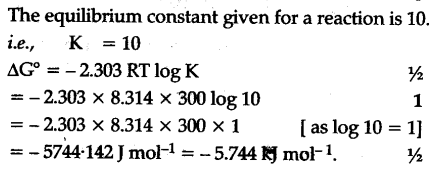# The equilibrium constant for a reaction is 10. What will be the value of \${{\Delta }_{0}}\$G° ? R = 8.314 \${{Jk}^{-1}}\$\${{mol}^{-1}}\$ = 300 K